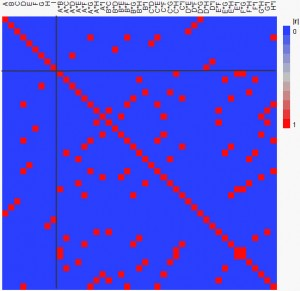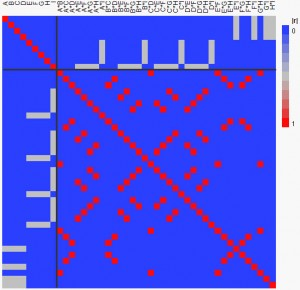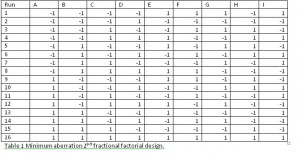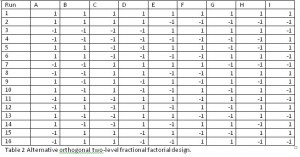Our World Statistics Day conversations have been a great reminder of how much statistics can inform our lives. Do you have an example of how statistics has made a difference in your life? Share your story with the Community!
Choose Language Hide Translation Bar
Comparing screening experiments using correlation cell plots

What is a correlation cell plot?

In my previous post, I proposed a new graphic diagnostic tool for evaluating designed experiments. The suggested graph is a cell plot showing the pairwise correlation between two model terms as a colored square. If, as in Figure 1, there are 45 terms in the model, then the cell plot created using JMP shows 45*45 = 2025 colored cells.What does this plot tell me?

Absolute correlations go from zero (blue) to one (red). The diagonal line of cells is red for any design, but it is most desirable for all the off-diagonal cells to be blue or at least on the blue end of the color spectrum. Every cell in Figure 1 is either pure blue or red. Cells colored pure red indicate that the associated model terms are indistinguishable from one another. In such a case, we say that the two effects are confounded. Looking at the first row of cells, we can see that the main effect of factor A is confounded with the D*G two-factor interaction.

The design shown in Table 1 below and graphed in Figure 1 is a regular fractional factorial design for nine factors in 16 runs. One way to construct such a design is to start with a full factorial design – in this case having four factors and 16 runs. Then, factor E is generated by the element-wise multiplication of the columns for factors C and D. Similarly, F = BD, G = AD, H = ABC, and I = ABCD. The result of this procedure is a design that is orthogonal for the main effects but which confounds 12 of the 36 two-factor interactions with a main effect. Suppose you ran this experiment and observed large effects associated with factors A, D and G. You would like to say that these three factors are all active. However, an equally explanatory model is the one with the terms D, G and D*G. So, the confounding in the design produces ambiguous analytical results. The only data-driven way to resolve such ambiguity is to do further experimentation.

Is there some way to avoid confounding main effects and two-factor interactions?

Figure 2 shows our diagnostic plot for one of the 87 nonisomorphic orthogonal fractional factorial designs for nine factors in 16 runs (see Table 2). Unlike Figure 1, the upper right section of Figure 2 has no red squares. Thus, no main effect is confounded with any two-factor interaction. Moreover, the largest absolute correlation of any main effect with any two-factor interaction is only one-half. On the negative side, this design has more interactions correlated with every main effect even though the correlations are not as large.Let us go back to the scenario we considered before. Can we distinguish between the model having the main effects A, D and G and the alternative model having the main-effects D and G plus the D*G two-factor interaction? Absolutely! This is true as long as the observed effects are sufficiently larger than their standard errors.

What is the bottom line?

Comparing Figures 1 and 2 side by side provides a quick way of assessing the relative merits of the two designs. I prefer designs where the diagnostic plot contains fewer pure red squares.

Design TablesArticle Labels

There are no labels assigned to this post.

Article Tags
1 Comment
Visitor

Why definitive screening designs are definitive wrote:

[...] my two previous posts, I introduced the correlation cell plot for design evaluation and then showed how to use the plot to compare designs. Here, I want to use the same plot to show why definitive screening designs are, well, [...]